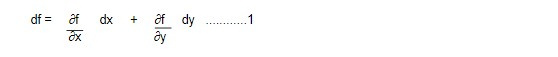# Legrendre Transformation

In Lagrangian formulation,

The partial derivative of L with respect to qi means a derivative taken with all q's and q̇ 's constant. But in Hamilton's principle (Hamilton's approach) q's and p's are equal status and Hamiltonian can be expressed as

H = H(qi, pi, t)

The transition from Lagrangian to Hamilton's formulation corresponding to change the variable from (q, q̇, t) to (q,p,t) where p is related to q and q̇ by the equation

pi = ∂ L (qi, pi, t)

The transition from Lagrangian to Hamilton's formulation corresponding to change the variable from (q, q̇, t) to (q,p,t) where p is related to q and q̇ by the equation.

pi = ∂ L (qj, pj, t)/∂q̇ i

where j index shows that the set of q's and q̇'s. The procedure for switching variable in this manner is provided by the Legendre transformation.

The Legendre transformation is a mathematical tool in classical mechanics and thermodynamics that maps a function of one set of variables to another function of a different set of variables, while preserving the relationships between the variables. It is used to obtain the thermodynamic potentials from the internal energy of a system, and vice versa.

In thermodynamics, the Legendre transformation allows one to switch from the internal energy representation of a system to the free energy representation, which can simplify the calculation of important quantities like entropy and pressure.

In classical mechanics, it can be used to convert the Lagrangian, which describes the system's dynamics, into the Hamiltonian, which provides a different perspective on the same system and may be more convenient for certain calculations.

Derivation of Legendre transformation

Let us consider a function f with the two variables x and y i.e f = f(x,y)

Thendf = udx + vdy ......2

where u = ∂f/∂x and v = ∂f/∂y ......3

We change the basis of description from x and y to a new distinct set of variables u and y so that the differential quantities are expressed in terms of du and dy.

Let g be a function of u and y defined by the relation

g = f - ux........4

dg = df - udx - xdu

or, dg = udx + vdy - udx - xdu

or, dg = vdy - xdu ......5

Differentiating with respect to y to equation 5, we get

∂g/ ∂y = v

v= ∂g/∂y .....6

Again differentiating with respect to u to equation 5 we get

∂g/ ∂u = -x

or, x = - ∂g/∂u

Hence x = - ∂g/∂u ........7

And v = ∂g/∂y .........8

which are analogous of equation 3.

Hence equation 8 are called Legendre Transformation equation

Let f(x,y) -> L ( q̇j , qj)

x = q̇j , y= qj

and u = ∂f/∂x = ∂L/ ∂ q̇j = Pj

=> Pj = ∂L/∂ q̇j = ∂f/∂x .....9

and g = f - ux

or, g = L - pjq̇j

=> g = - H

And v = ∂g /∂y = ∂f/∂y

v= - ∂H/∂qj = ∂L/∂qj = ṗj

=> ṗj = - ∂H/∂qj = ∂L/∂qj ..............10

Hence from Lagrangian ( L) and Hamiltonian ( H) we can find the pj and ṗj using equation 9 and 10.

Next topic: Cannonical transformation

This note is a part of the Physics Repository.